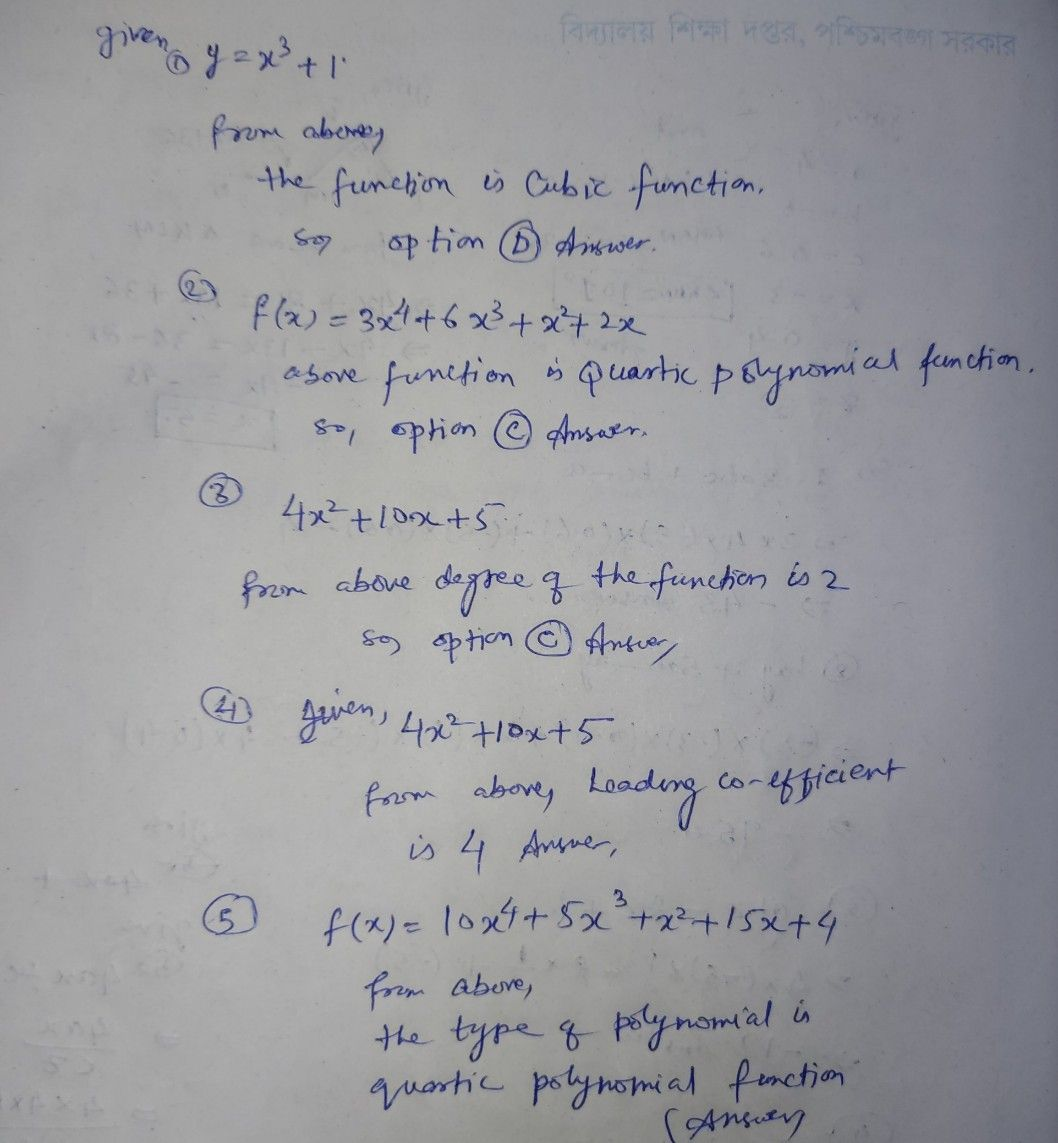Symbol
Problem1 What function is $y=x^{2}3+17$ A. Linear Function B. Quadratic Function C. Quartic Function D. Cubic function 2. What type of połynomial function is $f\left(x\right)=3x^{n}4+6x^{n}3+x^{n2+2x2}$ $A$ Cubic Polynomial Function B. Quadratic Polynomial Function $C$ C Quartic Polynomial Function D. Zero Polynomial Function 3. What is the degree of the function $4x^{A}2+10x+52$ $A0$ B. 1 $C.2$ $D.3$ $4$ Which is the leading coefficient of the function $4x^{n}2+10x+52$ $A-4$ $8-2$ C.O $D.2$ 5.What type of polynomial function is $f\left(x\right)=10x^{x}4+5x^{n}3+x^{n}2+15x+47$ $A$ Cubic Polynomial Function B. Quadratic Polynomial Function C. Quartic Połynomial Function $2a$ Zero Połynomial Function